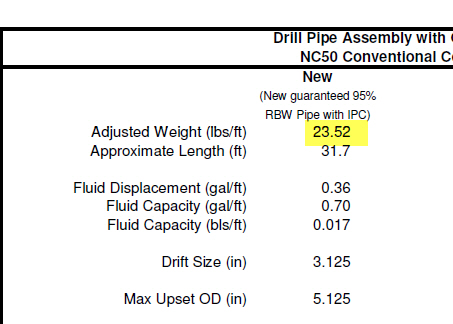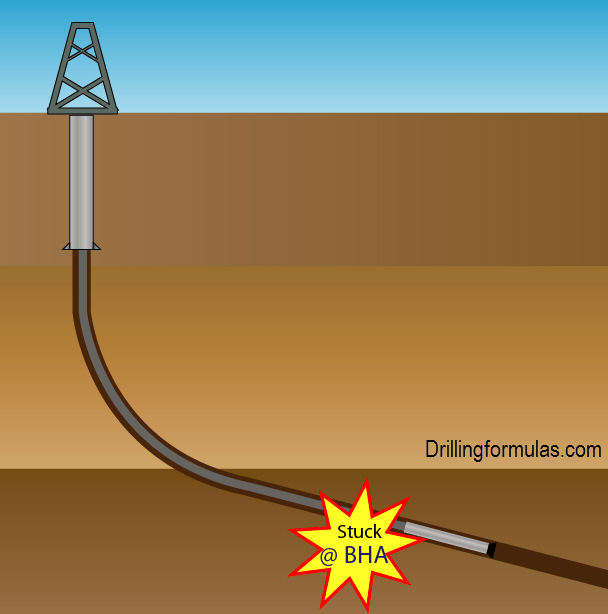# Determine Stuck Pipe Depth Based on Real Example

This is the real example of how to determine stuck depth using the stuck depth calculation.

Well Information:

• TD = 8,900’MD/5,600’ TVD
• Bit Size = 8.5”
• Drilling fluid = water based mud (PHPA system)
• Formation: sand shale sequence
• BHA: 7” mud motor + 9 stands of 5” HWDP S-135, 4-1/2” IF connection
• Drillstring: 5” DP S135, 4-1/2” IF connection
• Neutral weight @ 6,850’MD = 175 Klb

While tripping out of hole to 6,850’MD, observe over pull 40 Klb over pick up weight. Stop tripping out and attempt to go down no issue. Attempt to rotate, pipe is able to rotate at 30K ft-lb. Attempt to circulate, observe restricted flow.Figure 1 – Stuck While Pulling Out

Based on the information, we would expect the cutting load bed issue. We attempt to determine where the stuck point is using the stuck depth formula.

Stuck Depth (ft) = (735,294 x e x Drill Pipe Weight (ppf)) ÷ (Differential Pull, lb)

Where;

e = drill pipe stretch, inch

Pipe Stretch Information

Pull the stuck string to 300 Klb and the stretch measurement is 29.4 inch from the neutral point.

Pull the stuck string to 350 Klb and the stretch measurement is 49.2 inch from the neutral point.

So

Differential pull = 350,000 – 300,000 = 50,000 lb

e = 49.2 – 29.4 = 19.8 inch

Drill Pipe Weight (ppf) = 23.52 ppf adjusted weight

Note: The adjusted weight is used instead of the plain weight because it represents the whole string better than the plain weight.  The plain pipe weight can be used for tubular as casing or tubing which don’t have tool joints.Figure 2 – 5″DP S-135 Specification

Stuck Depth (ft) = (735,294 x 19.8 x 23.52) ÷ (50,000)

Stuck Depth (ft) = 6,784 ft

Based on this calculation, the drill string is stuck at the BHA.Figure 3 – Stuck Location (Stuck at BHA)

Note: This stuck pipe formulas is derived from Young’s Modulus equation therefore it does not account for friction in the wellbore. Using this formula will give you rough idea where the stuck pipe is and this is a good location for you to start determine the stuck depth using a wireline free point tool. The precision location must come from the free point tool only.

Reference book:Formulas and Calculations for Drilling, Production and Workover, Second Edition

Share the joyWorking in the oil field and loving to share knowledge.

### 5 Responses to Determine Stuck Pipe Depth Based on Real Example

1.Rury says:

is it the neutral point means weight of the string before the over pull increased?

how about if we use, weight of string after stuck – weight of string at neutral point?
also measured the stretch of the string from neutral point?

so from the equations,
Weigth of Neutral points =175 klbs
Weight of pull stuck string = 300 klbs

e=29.4 (stretch from neutral point)

so we got : (735,294×29.4×23.52) : (300,000-175,000)

= 4067,5 ft

above the BHA?

2.wiliam says:

how to measure e – where is neutral point?

3.Jeffry Syam says:

My calculator says: 6,848′ (2 feet above bit)

4.Khalil says:

According to my knowlege this formula is applied only in verical holes only. Am i right?

•DrillingFormulas.Com says:

It can be used in a deviation well too but it will give you rough idea only. The result is a good start before running the free point tool. This formula does not account for the friction so the error increases with deviation since more deviation creates more friction.

This site uses Akismet to reduce spam. Learn how your comment data is processed.